# Explain The Logic EX-OR Gate (Exclusive-OR Gate) and Its Operation With Truth Table

## EX-OR Gate (Exclusive-OR Gate)

Two special logic circuits that occur quite often in digital systems are the exclusive-OR and exclusive-NOR circuits.

The circuit of an exclusive-OR gate is shown in fig 1 .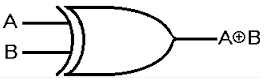Fig 1

As obvious, there are two inputs and one output. The exclusive-OR operation is denoted by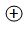. Hence the expression for the output of the circuit is given as :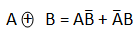### Truth Table of EX-OR Gate

The truth table for two input  EX-OR gate is shown in Table 1 .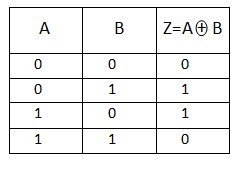Table 1

The output expression for two input EX-OR gate, in short, is given as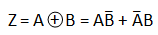This circuit is also called an inequality comparator or detector because it produces output only when the two inputs are different.

The main characteristic property of an EX-OR gate is that it can perform modulo-2 addition. It should be noted that the same EX-OR truth table applies when adding two binary digits (bits). A 2-input EX-OR circuit is therefore sometimes called a modulo-2 adder or a half adder. The name half adder refers to the fact that possible carry-bit, resulting from an addition of two preceding bits, has not been taken into account. The name Exclusive-OR is derived from the fact that its output is a 1, only when exclusively one f its input is a 1 (it excludes the condition when both the inputs are 1).

### Ex-OR Gate Equivalent Circuit

The Ex-OR function is not a basic logic gate but a combination of different logic gates connected together. Using the 2-input truth table above, we can expand the Ex-OR function to: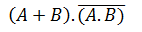which means that we can realise this new expression using the following individual gates.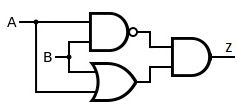Fig 2

One of the main disadvantages of implementing the Ex-OR function above is that it contains three different types logic gates OR, NAND and AND within its design.

One easier way of producing the Ex-OR function from a single gate is to use the universal gates NAND gate or NOR gates as shown below.

### Ex-OR Function Realisation using NAND gatesFig 3

### Ex-OR Function Realisation using NOR gates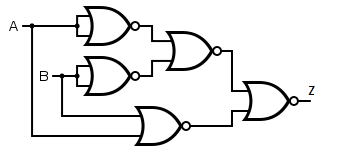Fig 4

### Standard Package

Fig 5 shows the pinout diagram of an IC7486 which is a quad 2-input EX-OR gate.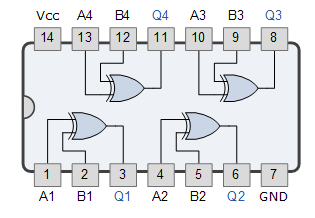Fig 5

It contains four 2-input EX-OR gate in a 14-pin DIP.

### Pulsed Operation

The pulsed operation of an EX-OR gate is illustrated in fig 6 .Fig 6

During time intervals  t1  input A is high but input B is low and during time interval t3 input A is low and input B is high, therefore output is high during these two time intervals. During time intervals t0 and t2, both inputs are low, hence output is low. And during time interval t4  both inputs are high, therefore output is low.